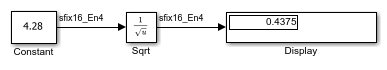# rSqrt of Fixed-Point Inputs

This example shows how to compute the rSqrt of a fixed-point input signal. The Sqrt block has the following settings:

• Method = `Newton-Raphson`

• Number of iterations = `1`

• Intermediate results data type = `Inherit: Inherit from input`

After one iteration of the Newton-Raphson algorithm, the block output is within 0.0459 of the final value (0.4834).

```ans = Simulink.SimulationOutput: tout: [51x1 double] SimulationMetadata: [1x1 Simulink.SimulationMetadata] ErrorMessage: [0x0 char] ```